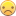## Subtracting dates in WHERE step of PROC SQL

Hi,

I'd like to run a Proc SQL step to link 3 databases.

I'm trying to use the WHERE statement to filter results by subtracting a date one from table from the other.

Here's what it looks like right now:

``````proc sql;
connect to oracle;
create table MRSA as select * from connection to oracle
(
select distinct
c.MRN,
a.account_num, a.discharge_date,
from table1 a,
table2 b,
table3 c
where a.td_patient_id=b.td_patient_id and
b.td_patient_id=c.td_patient_id and
0 <= (datepart(b.collect_date) - datepart(a.admit_date)) <= 2 and
);
disconnect from oracle;
quit;``````

I'm receiving this error when running:

ORACLE prepare error
ORA-00933: SQL command not properly ended

Thanks for the help.

1 ACCEPTED SOLUTION

Accepted SolutionsPGStats
Opal | Level 21

## Re: Subtracting dates in WHERE step of PROC SQL

Try replacing

``0 <= (trunc(b.collect_date, 'DDD') - trunc(a.admit_date, 'DDD')) <= 2``

with

(trunc(b.collect_date, 'DDD') - trunc(a.admit_date, 'DDD')) >= 0 and
(trunc(b.collect_date, 'DDD') - trunc(a.admit_date, 'DDD')) <= 2

then try using between 0 and 2

PG
6 REPLIES 6

## Re: Subtracting dates in WHERE step of PROC SQL

Is that the correct usage of DATEPART() function in Oracle?

## Re: Subtracting dates in WHERE step of PROC SQL

Ah good point.  I tried using the trunc function instead but got the same error``````proc sql;
connect to oracle;
create table MRSA as select * from connection to oracle
(
select distinct
c.MRN,
a.account_num, a.discharge_date,
from table1 a,
table2 b,
table3 c
where a.td_patient_id=b.td_patient_id and
b.td_patient_id=c.td_patient_id and
0 <= (trunc(b.collect_date, 'DDD') - trunc(a.admit_date, 'DDD')) <= 2 and
);
disconnect from oracle;
quit;``````

## Re: Subtracting dates in WHERE step of PROC SQL

To narrow down the cause of the error, I would try to remove more and more parts of the query (beginning with the WHERE clause) until the error disappears. Then reconstruct the original query in even smaller steps, until the error reappears. (If it doesn't reappear, you're lucky.) Of course, you have to make sure that each of the reduced queries still makes sense.

I think, at least one simplification could be done anyway: Aren't the last two lines of the WHERE clause equivalent to trunc(...)='...' or is Oracle's logic at odds with mathematics?

## Re: Subtracting dates in WHERE step of PROC SQL

I QC'd and the cause of the error is this line:

``0 <= (trunc(b.collect_date, 'DDD') - trunc(a.admit_date, 'DDD')) <= 2``

When I comment it out, the code runs.

For the last two lines, I changed it just for QC purposes.  The actual date range to pull the data will be over a couple of years.

## Re: Subtracting dates in WHERE step of PROC SQL

Good. And, in general, Oracle can handle such chains of inequalities (as it is possible in SAS, except in SAS Macro language)?

Maybe you could try to use the BETWEEN condition trunc(...) - trunc(...) between 0 and 2 instead (if that is the correct syntax in Oracle). Or something like abs(trunc(...) - trunc(...) - 1) <= 1 (assuming there is an ABS function like the one in SAS).PGStats
Opal | Level 21

## Re: Subtracting dates in WHERE step of PROC SQL

Try replacing

``0 <= (trunc(b.collect_date, 'DDD') - trunc(a.admit_date, 'DDD')) <= 2``

with

(trunc(b.collect_date, 'DDD') - trunc(a.admit_date, 'DDD')) >= 0 and
(trunc(b.collect_date, 'DDD') - trunc(a.admit_date, 'DDD')) <= 2

then try using between 0 and 2

PG
Discussion stats
• 6 replies
• 3557 views
• 0 likes
• 4 in conversation